## Estimating Mash pH

As having proper mash pH is as important as having proper mash temeperature in terms of the quality of the beer that is produced from the mash brewers naturally seek a means of controlling that pH. It would certainly be of great benefit if there were some spreadsheet or calculator into which a brewer could insert the characteristics of his water and malts and which would calculate the resulting pH of the mash. Intuition says that this should be possible and there are, in fact, several such calculators and spreadsheets available which purport to do it. The writer advocates experiment by which he means that it is absolutely fine to use a calculation to 'ball park' the expected pH but ultimate reliance should be placed on a properly made measurement using a good quality pH meter.

Predicting mash pH is a difficult thing to do – not so much because the model is complicated as because it is difficult to get reliable data to put into it. At the same time while it is fair to say that the model is simple it is only so to engineers and scientists who are familiar with similar models and are well practiced at using them to solve engineering problems. Our task here is to try to explain the model and how to use it in terms that will be understandable to readers without a lot of technical background. One way to do this is not to explain the model at all – to simply prescribe a set of steps to follow which the reader can do without understanding the reasons behind any of the steps. We will do that but only after the model is, in fact, explained for the benefit of those who do have some technical background. Our goal would be to have them understand why the various steps in the algorithm are necessary in which case going through the algorithm, especially with numerical examples, will serve to illustrate the concepts. Other readers can simply follow the prescription as a prescription. They will be able to get answers even if they do not understand the details.

The Model

We have defined an acid as something which donates protons and a base as something which absorbs them. A mash is a mix of acids and bases and they interchange protons until a pH is reached at which the sum of all the protons donated by acids exactly matches the sum of all the protons absorbed. The problem is ‘simply’ one of bookkeeping but that is not such a simple task as there are several things in a mash which absorb or donate protons and each must be accounted for. These are

1.     The water most probably contains some bicarbonate ion. These can either donate or absorb protons depending on the direction of pH shift. Bicarbonate is thus simultaneously an acid and a base (said to be amphoteric)

2.     The water may contain carbonate ions though typically in relatively small quantity. Carbonate is a proton absorber.

3.     The brewer may add some bicarbonate or carbonate to raise pH (absorb protons) where dark malts are used.  Both ions are bases in this role.

4.     The water may contain, or the brewer may add, calcium and/or magnesium ions. These react with malt inorganic phosphate to release protons. Calcium and magnesium are, thus, effectively acids when phosphate is present.

5.     The brewer may add strong acids such as hydrochloric, sulfuric or lactic acids to a mash to lower its pH.

6.     The brewer may add weak acids (such as phosphoric acid) to lower mash pH.

7.     Base (pale malts) will, unless furnished with protons, settle in at pH’s higher than desired. In a mash with properly adjusted pH base malts are, thus, proton absorbers i.e. bases.

8.     Darker (crystal, caramel, roast…) malts will, unless their protons are absorbed, generally settle in at pH lower than desired. In a properly pH adjusted mash these malts are thus proton donors i.e. acids.

In a mash made with multiple malts there may be almost a dozen items to model in terms of their proton donating or absorbing capacities. As noted above we seek the value of pH which makes the total donations equal the total acceptance. Although we did not say so earlier it is probably clear that this has to be done by guessing. In other words there is no formulas that we can plug numbers into that gives us the pH of the mash. But there are formulas for each component of the mash into which we can plug a hypothecated pH and which will tell us how many protons are released or absorbed by the particular component at that pH. The number of protons absorbed or released will, in nearly all cases, be a function of the hypothecated pH but it may be a function of other things as well, for example, in the case of water, the pH at which the water comes to us.

In the following sections we will explore the proton donation models for the various components we listed above

Bicarbonate in the Source Water

Bicarbonates in the source water are, on the one hand, the most complicated proton source/sink to model but that model is the most accurate of any of the others. We discussed in Chapter X how carbonic acid donates a proton to become a bicarbonate ion and how a bicarbonate ion donates a proton to become a carbonate ion: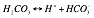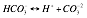We have also noted that in the first equation the ratio of the concentration of bicarbonate ion to carbonic acid molecule isand similarly, in the second, the ratio of carbonate to bicarbonate is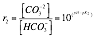where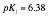and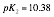are the two acid constants of, respectively, carbonic acid and bicarbonate ion.

Now let us suppose that the concentration of carbonic acid is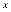mmol/L. Clearly, then, the concentration of bicarbonate ion will be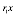and of carbonic,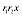. The total of carbonic acid molecules, bicarbonate ions and carbonate ions in this solution is clearly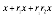and the fraction of this total which is carbonic isThe fraction which is bicarbonate is then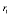time this orand the fraction which is carbonate is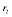time that or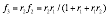Clearly, if there is 1 mmol of carbo (carbonic plus bicarbonate plus carbonate i.e. 1 mmol of carbon) the three fractions give the amounts of each of the three species and the electrical charge on the 1 mmol is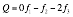because carbonic is electrically neutral, bicarbonate has a charge of -1 and carbonate a charge of -2. If there is a total carbo content of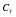mmol then the total charge on the carbo is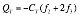This charge depends only on the amount of carb and the pH (plus, of course, the two acid constants. This makes it possible to prepare a graph like this one:which shows the charge on 1 mmol of carbo.  If we consider a sample of water with 1 mmol carbo per liter which comes to us at pH 7.6 the charge on the bicarbonate and carbonate ions is, according to this plot, -0.95. If we were to use this water to make a mash which we brought to pH 5.4 the charge on the bicarbonate and carbonate in each liter of the water would have been reduced to -0.1. To reduce negative electric charge from -0.95 to -0.10 mmol (mEq) clearly requires that 0.85 mmol (mEq) of positive charges (protons) be supplied from some source. This could be bottle acid or an acid malt. At this point we are only concerned with the amount of acid (protons) or base (proton absortion) that is required to effect a pH change in the brewing water. This curve, or the calculations implied by the formulas above allow us to calculate that.

For water samples with carbo at levelthe charge amounts and differences as taken from the curve or formulas are simply multiplied by. That brings us to the question “How do we determinefor a water sample?”

Determining- Alkalinityis a function of the alkalinity of the water sample, its pH and the pH which defines the alkalinity (the end point pH in the alkalinity titration). The same curve or formula set is used. If we are given a sample of water at pH 7.6 the charge per mmol carbo per liter is, as above, -0.85 mEq per liter. If we titrate to pH 4.3 (marked on the curve) we see that the charge is reduced in magnitude to about -0.01. It would, thus, require 0.84 mEq of acid per liter to effect this pH change. Since the alkalinity is defined as the amount of acid required to decrease sample pH to a specified end point the alkalinity of this water is 0.84 mEq/L if it contains 1 mmol/L carbo. Suppose, though, that the alkalinity of the water sample is some other value such as 2 mEq/L (100 ppm as CaCO3) or, put another way, that rather than add 0.84 mEq of acid to a liter of the sample to reach pH 4.3 we had to use 2.00 mEq. Clearly, then, the amount of carbo is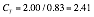mmol/L.

This is an approximation as, in the first place, we are reading charge numbers from a graph. Another factor which contributes to the approximate nature of this result is that it ignores the fact that some acid is required to move the pH of even deionized water from pH 7 to pH 4.3 or, in other words, even DI water has a small alkalinity. Ignoring  this means that we slightly over estimatewhen using this method. Spreadsheet, such as the one at wetnewf.org, account for this.

Approximate values for CT can be obtained from the following graph. Visually interpolate between the pair of curves lines between which the intersection of pH and alkalinity lie. For example, a water with alkalinity 120 and pH 7 is seen to have total cargo content of about 2.9 mmol/L.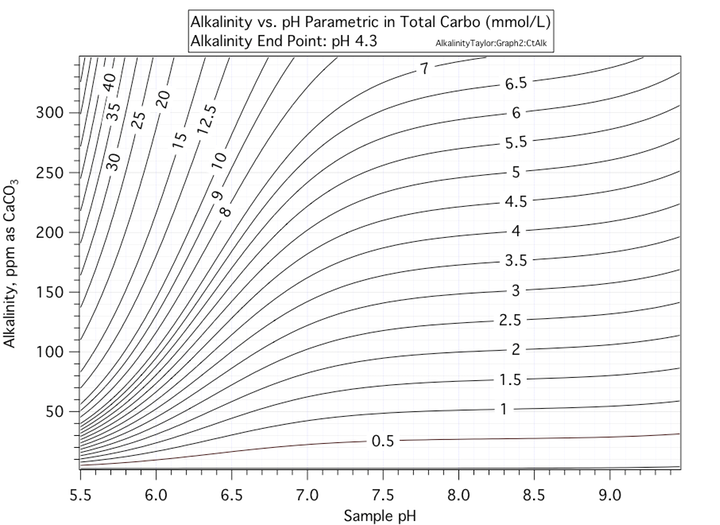If we add bicarbonate to the mash we use the same curve or formula but, in this case, the result (proton absorption) depends only on the final pH. This is because the initial effective pH is always the same – close to 8.3. When we add bicarbonate ion to solution we are adding pure bicarbonate ion – no carbonic molecule or carbonate ion. The fraction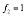and the other fractions each equal 0. If there is no other source or sink of protons (i.e. if bicarbonate is added to DI water) there will be a slight but finite redistribution. Some of the bicarbonate will convert to carbonic and an equal amount to carbonate. This does not result in a redistribution of charge. For that to occur there would have to be some source or sink of protons and in DI water there is no such source or sink.so that the total charge is -1 mEq/mmol bicarbonate added. Our task is, as before, to find out what the charge will be at the hypothesized mash pH. Using the same value of mash pH as above the charge on the added bicarbonate will be -0.1 mEq/mmol bicarbonate added and the amount of acid necessary to effect the change of added bicarbonate powder to solution at pH 5.4 is (-0.1 - -1.0) = 0.9 mEq/mmol added.

We don’t generally advocate the use of carbonate unless it is sodium carbonate and this is because calcium carbonate is slow to dissolve and because where phosphate is present (as it is in mash) phosphate coalesces with calcium resulting in the release of protons. Much of the proton absorbing capacity of the carbonate ion is consumed by the precipitating calcium. Only about 30% is available to absorb protons from other sources. This is not a factor with sodium carbonate.

We include discussion of carbonate mostly for completeness and note that the concept is the same as for bicarbonate except that the charge on 1 mmol of carbonate ion is -2 mEq (as opposed to -1 for bicarbonate). Thus if we added 1 mmol of carbonate ion to the same mash that we have been discussing, that is a mash which we will bring to pH 5.4, we have a proton requirement for the carbonate equal to (-0.1 - -2.0)  = 1.9 mEq/mmol as opposed to 0.9 mEq/mmol of bicarbonate

As noted before phosphoric acid will, if the pH is high enough, in the presence of calcium strip calcium out of the mash and in so doing release extra protons. At mash pH it takes a lot of calcium to reach the threshold amount. It is impossible to accurately model the interaction between added phosphoric acid, inorganic phosphate in the malt and calcium in or added to the water or mash. Here we take the approach of assuming that we are below phosphate saturation.

Phosphate is actually modeled in exactly the same way as bicarbonate except that as phosphoric acid is a triprotic acid there are three acid constants: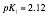,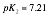and. These result in 3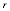factors and 4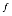factors with the first being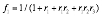and the others found by multiplying eachby the like numberedjust as was done for the bicarbonate fractions. Similarly to what we did for bicarbonate we can compute the total charge on a mmol of phospo as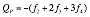and, also as we did for bicarbonate, plot this charge:When we add phosphoric acid to a solution we are adding the uncharged acid. If the pH comes to pH 5.4 the graph shows that the charge will be -1.02 mEq/mmol acid added. This means that the acid yielded up 1.02 mEq of protons per mmol in going to pH 5.4.

Malts

The malts contain a mixture of acids. If we knew amounts and pK values for each acid we could make curves like the one we just did for phosphoric. As malt contains a lot of phosphate the phosphate curve would be a major contributor to proton acceptance/donation picture for malts. As we don’t know all the details, however, we cannot make such a computation for malt and must use the more practical approach of mixing a sample of the malt with distilled water, measuring the pH, adding some acid, measuring the pH, adding some base, measuring the pH and so on until we have enough data to construct a curve (or, in actuality, the mathematical model of a curve) like the ones in the figure below: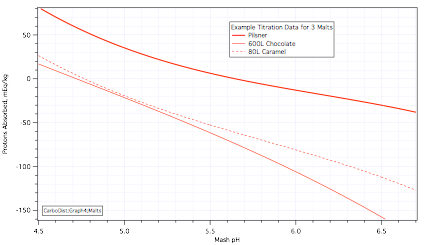These curves are similar to the previous graphs but the vertical axis is different in two ways. First, it is not calibrated in terms of charge but rather in terms of protons absorbed. Second, the protons absorbed are per killogram of the malt (not per millimole of acid or carbo as is the previous graphs). They are used in the same way as the previous graphs, however. If, for example, we were to make a beer with the Pilsner malt whose characteristics are described in the uppermost trace and realize a mash pH of 5.4 (same as for all the other examples) the Pils curve says that each kg of the malt would absorb 11.15 mEq protons or, put another way, that we would have to supply 11.15 mEq protons to each kg of this malt in order to lower its pH to 5.4. The Pils curve also shows us that if we supply 0 mEq protons, in other words, if we mash this malt with distilled water, that the pH of the mash will be 5.65. Note that these curves are for room temperature pH.

Now look at the curve for the 80L Caramel malt. At pH 5.4 it indicates that -46.6 mEq are absorbed by each kilogram. As negative absorption is donation we are really saying that each kilogram of this this malt, brought to pH 5.4 releases 46.6 mEq of protons. Clearly this caramel malt can be used to supply some or all of the protons required to lower the base malt pH. It can also be used to supply some of the protons required to overcome water alkalinity. Note that this curve intersects the 0 proton axis at pH 4.77 and that is its distilled water mash pH.

The curve for the 600L Chocolate malt is similar to the Caramel malt curve and, as is well known, roast malts can be used to supply needed mash protons.

Each malt is modeled by an equation in the following form: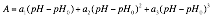in whichis the proton absorption in mEq/kg,is the pH of the mash,is the distilled water pH of the particular malt and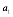etc. are coefficients which, like, depend on the particular malt. There can be fewer than 3 coefficients and in some cases more may be necessary but generally speaking each malt can be described by 4 numbers:and 3 coefficients. Clearly it is easier and more accurate to enter this formula into a spreadsheet than it is to read numbers off the curves but as we have shown, reading them off the curves is doable.

There are several warnings that go with these malt curves. First is that there will be variation from batch to batch, from maltster to maltster, from farmer to farmer and from cultivar to cultivar. In addition to those variations (of unknown magnitude) we must consider moisture content of a grain as proton absorbing and donation abilities are per unit weight and moisture is part of weight. Finally, the amount of acid absorbed or given off depends on time, temperature, coarseness of grind and mixing. The curves given above are curves for very finely ground malt 25 minutes after strike. The equivalent curves for 20 minutes and 30 minutes are not the same. This problem alone, of course, makes mash pH prediction very difficult as one cannot simply ask ‘What is mash pH’ but must in fact specify a time at which he wants to know mash pH.

See Measuring Malt Parameters below for more details on how malt data are obtained and for more detail on the mathematical model.

Calcium and Magnesium

The chemistry of phosphates, calcium and magnesium is quite complex – beyond what we can reasonably model. But we can take Kolbach’s definition of residual alkalinity, the amount of alkalinity, expressed in mEq/L, not cancelled by the metal/phosphate reaction, which definition is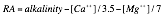in which the bracketed quantities in brackets are the concentrations of those two ions, to indicate that there is a proton release of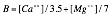mEq/L given the respective calcium and magnesium ions. This is very simple and probably not terribly accurate but as the protons from this source usually have small effect compared to the protons from other sources (added acids for example) and as there is no practical alternative we will use this approximation. Note that the value does not depend on pH and this is counter intuitive. We can say, however, that it probably does apply in the narrow range of pH that we consider acceptable for mash.

Added Strong Acids and Bases

We have covered weak acids and bases in the discussions of phosphate and bicarbonate. Strong acids and bases (sulfuric, hydrochloric, lactic acids, sodium hydroxide, potassium hydroxide) are much simpler. The exception is calcium hydroxide which, while it is a strong base which releases all its hydroxyl ions also contains calcium which reacts with mash phosphates thus releasing protons which immediately react with the hydroxyl ions. As with calcium carbonate, calcium hydroxide seems to exhibit about 30% of what its proton absorbing capacity ought to be.

Putting that exception aside: 1 mEq of strong acid donates 1 mEq of protons and 1 mEq of strong base absorbs 1 mEq of protons. Again this is ostensibly independent of pH and again this is because we are defining a strong acid or base as one that is completely, or nearly completely, dissociated at mash pH.

Example

At this point we have given a description of the models for proton donation/absorption for each of the components of the mash and we can turn to an example of two for purposes of illustration. Let us suppose that we wish to mash 50 kg of the Pilsner malt discussed earlier and 5 kg each of the chocolate and caramel malts with 150L of water with alkalinity of 4 mval (4 mEq/L, 200 ppm as CaCO3) which contains 4 mEq/L calcium hardness (also 100 ppm as CaCO3) and nothing else except 150 mmol (1 mmol/L) phosphoric acid which the brewer adds to combat the rather high alkalinituy of the water. The following table shows proton requirement predictions for each of the applicable proton requirement models for four sets of conditions. In the first set we ask the models for proton requirement predictions for pH 5.4. We see at the bottom of the first column that we would need 154 mEq more protons to reach 5.4 and that, therefore, the actual mash pH will be somewhat higher than 5.4.

 Mash pH 5.4 5.454 5.4 5.517 Water (150L) Alkalinity, ppm as CaCO3 200 200 200 200 Water pH 7 7 7 7 Water Ct, mmol/L 4.97 4.97 4.97 4.97 Water Proton Requirement, mEq 530.3 521.9 530.3 511.2 Calcium Proton Requirement, mEq −171.4 −171.4 −171.4 0 Phosphoric Acid, mmol 150 150 301.5 150 Phosphoric Acid Proton Requirement, mEq −152.2 −152.5 −305.9 −152.9 40 kg Pilsner Malt Proton Requirement, mEq 446.2 340.3 446.2 224.7 5 kg Chocolste Malt Proton Requirement, mEq −266.4 −288.9 −266.4 −315.0 5 kg Caramel Malt Proton Requirement, mEq −232.8 −249.4 −232.8 −267.9 Total Proton Requirement, mEq 153.7 0 0 0

With all the computations done in a spreadsheet it is a simple matter to enter trial values of pH to discover the pH that results in a 0 total proton requirement. The result, pH 5.454, requires fewer protons to lower water and Pilsner malt pH’s. At the same time the phosphoric acid releases more (though not appreciably more) protons as do the two dark malts. 5.454 is the predicted pH for this mash if we take no further steps.

In the third column we have decided that we want pH 5.4 and will make up the deficit of 154 mEq protons by adding additional phosphoric acid. As it appears that 1 mmol of phosphoric acid releases about 1 proton at mash pH (see earlier phosphoric acid curve) we simply add about 154 mmol more phosphoric acid. In fact, we set the pH to 5.4 and fiddle with the phosphoric acid number until 0 net requirement is realized. This is at 305.9 mmol total phosphoric acid (2 mmol/L).

In the final column we look at the effects of our calcium model by redoing the mash pH prediction without any (this would require that the entire alkalinity be balanced by sodium and or potassium which would be a bizarre situation in the real world). Earlier columns in the table show that we are estimating the calcium will produce about 171 mEq protons. Without those protons (last column) the pH which brings about 0 proton requirement is pH 5.517. This is to be compared to pH 5.454 and shows an increase of 0.063 pH. It is interesting to compare this to Kolbach’s 0.00168 pH per ppm as CaCO3 RA increase for mash pH. This is equivalent to 0.084 pH increase per mEq/L. In removing 4 mval calcium from the water we are taking out 4/3.5 = 1.143 mEq/L Residual Alkalinity. Kolbach would expect that to produce (1.143)(0.084) = 0.096 pH increase. Our result, 0.063 is less but then remember that Kolbach’s number is an average over a range of conditions that we don’t know.

Summary

Prediction of mash pH is relatively easy to do if one is comfortable with an assortment of models which predict the abilities of mash components to absorb and release protons. The largish number of these makes the problem a bookkeeping problem of magnitude enough that some readers may be confused. The models themselves are subject to various criticisms and getting data for them, in the case of malts, is difficult. For this reason mash pH predictions are not expected to be terribly accurate. The use of the models, especially as programmed into a spreadsheet, can be very instructive to brewers trying to better understand the ins and outs of this complicated subject. Readers are encouraged to try several example of their own with the spreadsheet. By doing so they will gain insight as to how the various numbers vary with pH and other malt and water parameters.

Measuring Malt Parameters

A buffer is a mix of acids, bases and salts that tend to hold a particular pH. For example the pH 7 buffer that we use to calibrate our pH meters is a mix of the mono basic and dibasic phosphate salts of either sodium or potassium. The term 'buffer' implies that the solution will absorb chemical shocks that try to shift the pH away from the buffer's design value. The degree to which a buffer resists pH shift is called its 'buffering power', 'buffering capacity', 'buffering potential' or just 'buffering'. There are a couple of ways to express this. For the present purpose we shall use milliequivalents of acid or base per unit pH change per unit mass. The more acid or base it takes to effect unit pH change the stiffer the buffer (the greater its buffering capacity).

Malt acts as a buffer. When water is added to it if the water is pure (DI) it tends to come to some pH and stay there until we add acid to it as we usually do because the DI mash (buffered pH) is usually higher than we want it to be. The buffering capacity of the malt and, of course, the amount of malt used, determines how much acid we must add.

At this point the discussion will be greatly enhanced by reference to the following graph. We will have more to say later about how it was obtained but for the present discussion it is sufficient to say that we took some malt, ground it up, put it in a metal beaker in a water bath, added DI water mixed with various amounts of acid and base and measured the pH 20 minutes after 'strike'. The open circles on the bottom half of the graph represent actual measurments which we know are in error from various causes. We therefore seek a simply described solid line which best 'fits' the data. The simple description of that line becomes our model for the behaviour of this malt when it is mashed.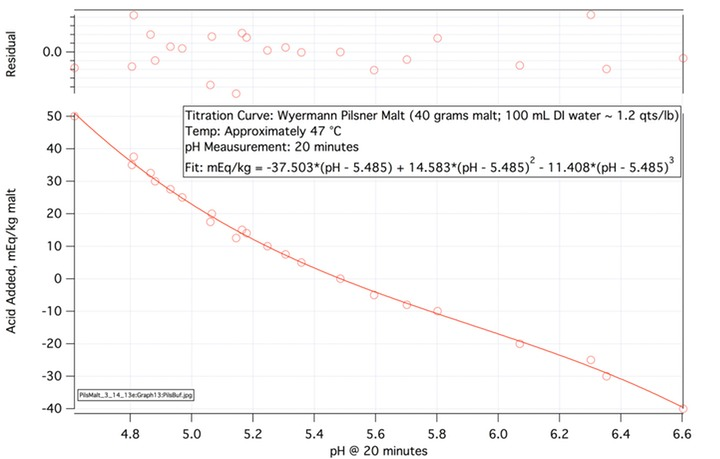Looking at the graph we see that when 0 acid was added to this malt the pH was 5.485 (you can't read the graph that accurately but that is what the pH meter read) . This is the DI mash pH of this malt at the approximately 47 °C mash temperature of these experiments. We usually like to work in terms of mash pH specified at room temperature but in this case we need to see what is actually happening in the mash and so took pH measurements at mash temperature. Using the general rule of thumb that measured mash pH increases about 0.0055 for each °C decrease in temperature we would add (47-20)*0.0055 = 0.1485 pH and conclude that the room temperature (20 °C) DI pH for this malt is 5.63 (that is low for a Pilsner malt but this was a fairly old sample - a point to which we will return).

If we decide that we want mash pH to be 5.2 (at protein rest temperature or 5.35 at room temperature) the graph shows that we would have to add 12 mEq for each kg of this particular malt we used. This is the alkalinity of the malt with repect to a pH shift from 5.48 to 5.2 (at mash temp). Alkalinity is the amount of acid which must be added to a system to reduce its pH to a specified value. Akalinity is not buffering or buffering capacity which is the slope of the curve (see Figure below). At pH 5.485 the buffering capacity of this malt is 37.5 mEq/kg-pH. The two are, of course related. The shift from pH 5.485 to pH 5.2 is -0.285 pH and multiplying this by the buffering at pH 5.485 gives -0.285*-37.503 = 10.7 mEq/kg which is close to the 12 that you would read off the graph. This is because in the region between 5.2 and 5.6 or so the curve is quite linear. It does not become non-linear until one is farly far from DI mash pH.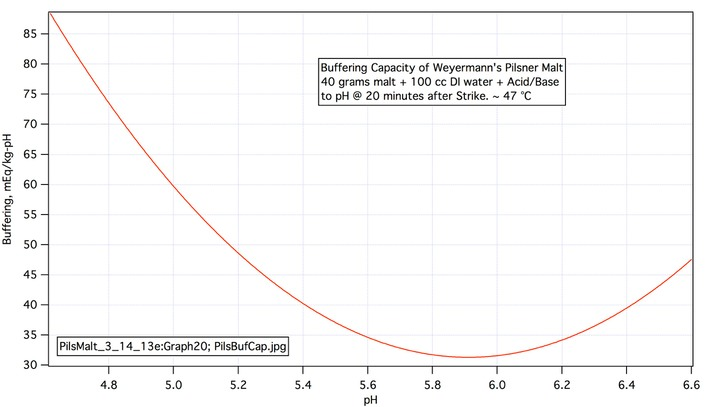Mathematical Representation of Titration Curve

We have already indicated that we could estimate the amount of acid required to lower the mash temperature pH of this malt to 5.2 by multiplying the difference between the desired and DI mash pH's by the slopw of the curve at the DI pH which, as we have indicated is -37.503 mEq/kg-pH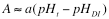Here A represents the amount of acid in milliquivalents per kg of the malt, a is the slope (-37.503 mEq/kg-pH) and the subscripts represent, respectively, the target (t) and distilled water (DI) mash pH's. This is fine for this base malt where the DI mash pH is close to the target i.e. where we may use the approximately linear nauture of the curve near the DI pH to advantage. But suppose we wish to use some colored malt whose DI mash pH is far from the target mash pH. It is not reasonable to assume that its titration curve would be any more linear than this base malt's curve and so the linear assumption probably won't suffice. Needless to say we would love to show you a titration curve for such a malt to illustrate this point but these curves are very time consuming to prepare. We do hope, eventually, to have at least one more posted here.

The non linearity is simply handled by adding additional terms thus: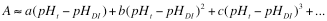Here b and c are simply additional coefficients. a is related to the slope (first derivative) of acid requirement with repspect to pH, b is related to the second derivative, c the third and so on. Some readers will recognize that this is a Taylor series expansion. As many terms as are necessary to follow the non linearity are used and this is determined by looking at the 'residuals' which are the differences between the data points and the model of them as represented by the formula for A. The coefficients a, b and c are found by a procedure which minimizes the sum of the square of the residuals. The residuals are plotted at the top of the graph. Enough terms have been taken when the residuals appear 'noisy' i.e. when no dependence of the residuals on pH is apparent. Three terms are sufficient here.

Before leaving the residuals lets take note that their rms is about 2 mEq/kg. This number can be used to give us a very rough idea as to the quality of the fit. If the slope of the curve is about 40 mEq/kg-pH it should be clear that the corresponding rms error in pH is approximately 0.2/40 = 0.05 pH.

Now let us define a set of functions distinguished from one another by the subscripts j: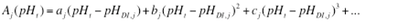j is the number of a malt in a grist we are interested in. Thus j =1 could represent the Pilsner malt we have been talking about withj = 2 could represent some Vienna malt or crystal malt with a separate set of parameters (and there could be more or less of them depending on how non linear these malts are), etc. To sumarize: Aj(pHt) is a formula into which one plugs a target pH and which computes from that the amount of acid you must add (or subtract - adding base is subtracting acid) per kiliogram of the malt used in order to atain the target pH per kilogram. We could, for example, program a bunch of Aj(pHt) into a spreadsheet - one cell for each.

Note that if we wish to work at room temperature pH all we have to do is decide how much of a pH shift there will be and add that to pHDI,j. If, for example, we think the offset is, as calculated above, 0.1485 we would simply use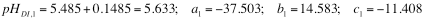as our model for this malt referred to room temperature (we note that we meausured this malt at 19.9°C at pH 5.601). The effect of this shift is a simple shift of the entire curve to the right by 0.145 pH.

The Problems

Readers who don't do this sort of thing frequently may be bewildered at this point but what we have described thus far is really very simple.  As we implied earlier there is a catch. In a nutshell, it is very difficult to get a set of a's, b's, and c's for malts. The reasons for this are several. In the first place we would have to consider that just because the bag says Weyermann's Pils on it does not mean that the bag you bought this spring is the same as the bag you bought last summer. Also there is little reason to suppose that either of them is the same as a bag of Pilsner malt obtained from a different maltster. In other words we have to be concerned about variabilities between maltsters, cultivars, farmers, seasons, age of the malt and its moisture content. Then there are difficulties in measuring the malt we have in hand. We will now turn to those and begin by sketching out the process we used in an effort to introduce as little error as possible.

Malt Titration

One cannot titrate malt in the way one titrates normal acids and bases because of the length of time it takes for reactions to take place. This depends on the coarseness of the grind (it takes liquid time to access the interior of larger grain particles) and on the fact that some of the reactions are just slow. Some investigators, including the writer, have tried to do titrations in the usual way. The writer found that this was not possible when he tried adding x, y and z units of acid, plotting the pH curve and then adding z, y and x units of base of equal strength. The data points from the acid base additions do not fall on the acid addition curve. Thus time from acid (or base) addition is a big factor. It can be controlled for by doin a separate one point 'titration' for each increment. Thus rather than weighing out 40 grams of malt, adding 100 grams of water, measuring the pH, adding 10 mEq/kg acid, measuring the pH, adding 20 mEq/kg, measuring the pH we have to weigh out 40 grams of malt, add 100 grams of water, measure the pH, throw away that mash, measure out 50 grams of malt, add 100 grams of water plus 10 mEq/kg acid, measure the pH, throw away that mash etc. This, of course, introduces errors with respect to meter calibration (as a lot of time passes), uniformity of sample grind, measurement of water and measurment of acid.

Our procedure was to grind 40 grams of malt to flour using a Waring spice grinder. Weigh out 40 grams with an analytical balance, place in a stainless steel beaker, measure out 100 mL DI water (using a TD cylinder), add the desired amoint of acid (H2SO4) or Base (NaOH), place the cylinder in a water bath at 50 °C, place buffer in the water bath, wait for the buffer mV reading to stabilize at around 48 °C buffer temperature, do the same with the other buffer, and wait for it to stabilize. While this is going on the water and acid (or base) are warming. Lower the metal beaker into the water bath and wait a few minutes. Now place the pH electrode in the beaker and pour in the water/acid. Stir and record pH for at least 30 minutes with occasional stirring throughout that period. Elecrode temperature and mV readings are requested from the meter every 5 seconds and recorded. The graph below shows a portion of one such recording and illustrates some of the difficulties with this approach.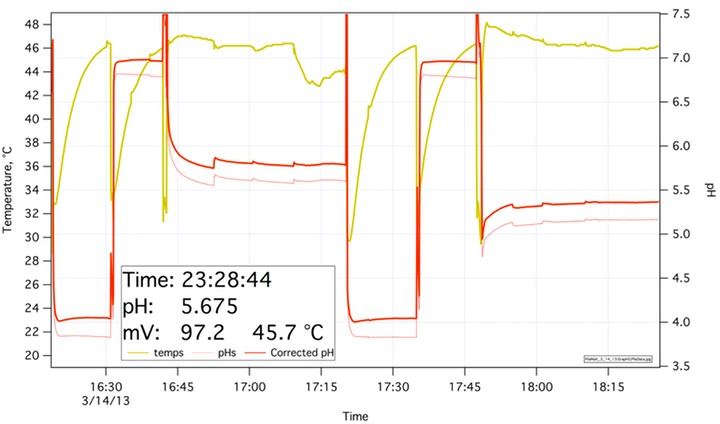To use the recording we start with the calibration period. Cursors are placed as late as possible on the portion of the red curve at 4 (about 16:30) and on the same curve at pH 7 (around 16:40). The computer finds the mV and temperature readings corresponding to those times, computes slope and offset and converts all mV readings to pH using those parameters and the temperature. Frequent calibration runs (there are two on this plot) and comparison of the slope and offset numbers show that calibration drift is small but we are working to 3 decimal places here and small drifts are enough to be a problem. Note the shape of the 'flat' parts of the calibration sections. It is clear from these that the electrode responds (even though it is old and has been abused by using it at these high temperatures) pretty quickly stepping from pH 4 to pH 7 in a minute or so but also note that it does not settle to its final reading for about 5 minutes. This is in part because the tempertaure is rising but it does take some time for the response of the electrode to go the last 0.02 or so of pH. Thus all the  data on the malt are 'convolved' with a finite duration electrode impulse response which broadens rapid changes in the plotted pH values. This cannot be dismissed and is an additional source of error.

The dashed gray curve is a plot of the value computed by the meter itself which I actually haven't calibrated for well over a year. Ignore it.

Referring to the plot: at the conclusion of the pH 7 cal run (around 16:40) water containing base was added to the grist. pH clearly zooms as the sodium hydroxide hits the electrode. Over a period of time the sodium hydroxide is absorbed by the grain and the pH begins to fall back. As the graph plainly shows he pH changes appreciably over the 30 minute period recorded. The discontinuities appear when the mash is stirred. This makes sense as, in the first place, stirring changes temperature and in the second place as it obviously has a big effect on the access of starch particles to liquor. These effects make it clear that the titration curve presented earlier will depend on how long after 'strike' the pH is measured. Or put another way, there is a different curve for each of several reasonable times. Which time should a pH prediction spreadsheet use? Clearly 5 minutes is not reasonable as things are very unstable then and clearly 40 minutes is not reasonable as that is close to the end of the mashing period in most cases. We chose 20 minutes as a time at which things are leveling off but obviously have the data for other times as well.

Note that there is another twist to this. In the second mash at the end of the second calibration (a little after 17:45) acid was added as opposed to base for the earlier one. This is indicated by a plummeting of the pH followed by a gradual rise. The implication is that acid additions and base additions approach equilibrium from different directions! How does this relate to what actually happens in the mash tun? There one has acids from dark malts, sauemalz etc and alkali from the water (unless low ion content water is used). Note also that stirring did not have as profound an effect as it did with the base addition.

Conclusions

1. The model for compution of required acid or base additions required to hit a particular mash pH or to predict mash pH when no acid or base is added is very simple.

2. Finding the parameters to put into the model is nigh on impossible. Days of lab work are required to get the parameters for a sigle malt sample.

3. Malt alkalinity and buffering are distinct and must not be confused.

3. Malt titration curves are not linear. Spreadsheets that assume they are incur error from so doing.

4. There is probably variation in malt models over sources, maltings, storage etc.

5. Time, mash temperature, stirring, nature of acid/base addition all have an effect on mash pH.

6. Given the above it is probably not possible to reliably estimate or set mash pH to better than ±0.1 or ±0.2 based on the use of a mathematical model. One can generally estimate that well based on experience without calculation.

7. For actual brewing, reliance should be placed on measurements made on test or actual mashes rather than on caculated values.

aj@wetnewf.org© A.J. deLange 2013